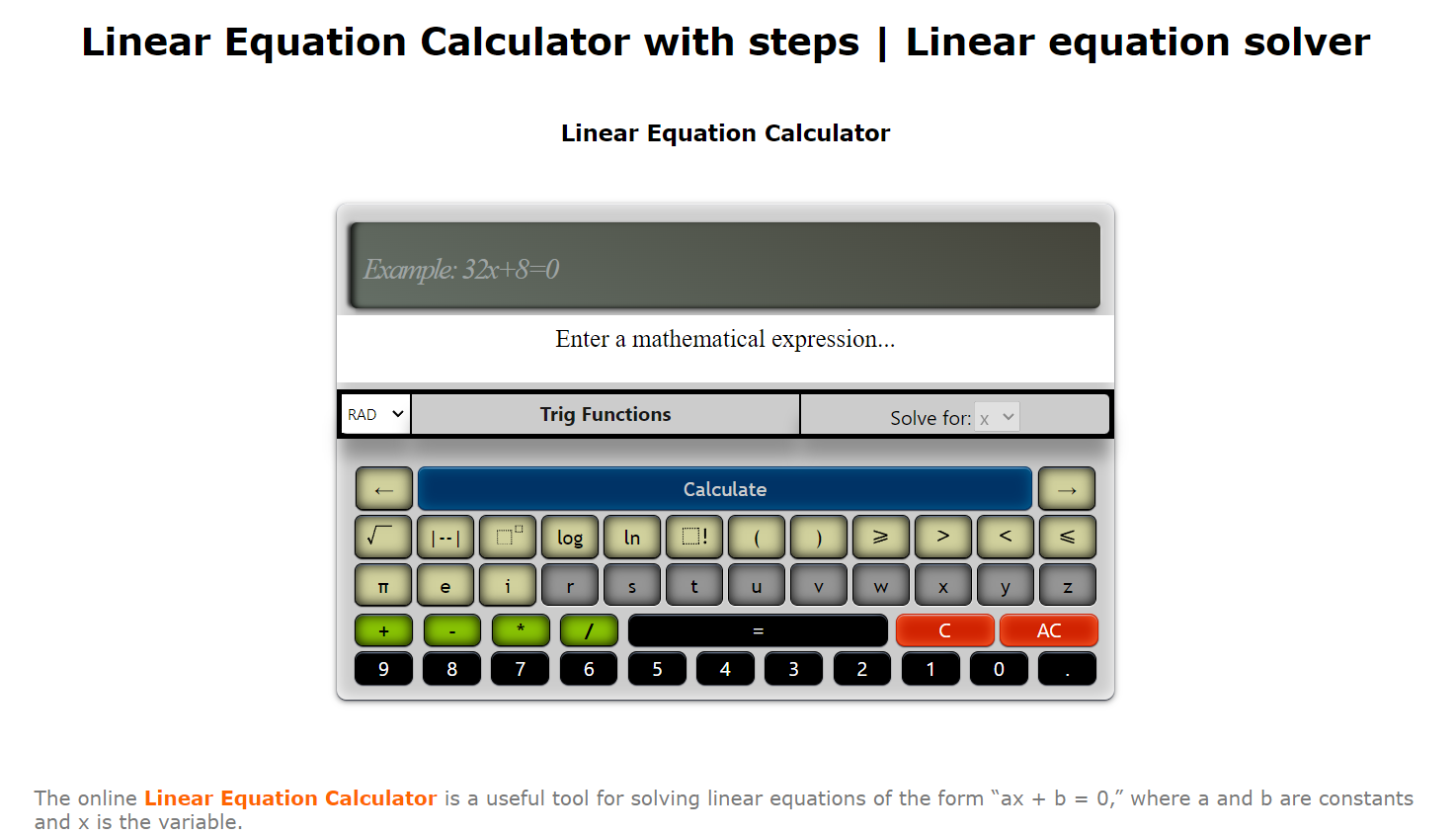What can I do?

99747 materialEducativo

textoFiltroFicha

# Linear Equations Calculator

tipo de documento Mathematics - Interactive activity

• I like 0
• Visits 24
• Save to
• Actions

The Linear Equations Calculator is easy to use and is ideal for students learning how to solve linear equations or for anyone needing to quickly and easily solve linear equations.

To use the Linear Equations Calculator you must perform the following steps:

1. Enter the linear equation. You can enter a linear equation with several unknown variables. Use only the functions and operators that are presented in Table 1.
2. If you have entered a linear equation with multiple variables, select the variable for which you want to solve the equation.
3. Press the “Calculate” button to get the solution explained step by step.

Linear Equation Calculator## Conceptual map: Linear Equations Calculator

Exclusive content for members of

D/i/d/a/c/t/a/l/i/aMira un ejemplo de lo que te pierdes

Categories:

Tags:

Fecha publicación: 26.12.2022

formatos disponibles

Comment

0

## Join Didactalia

### Browse among 99747 resources and 542519 people

Regístrate >

O conéctate a través de:

Si ya eres usuario, Inicia sesión

Do you want to access more educational content?